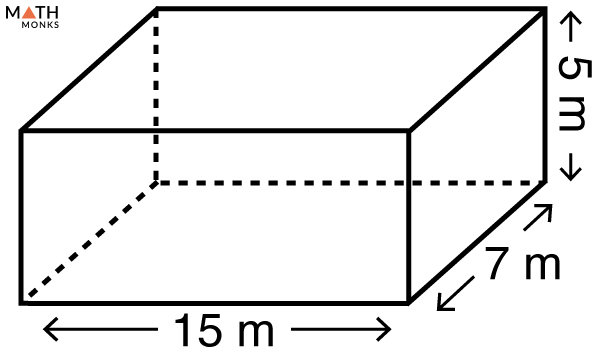# Surface Area of a Rectangular Prism

The surface area of a rectangular prism is the entire space occupied by its outer layer (or faces). It is expressed in square units such as m2, cm2, mm2, and in2.

## Formulas

Like all 3-dimensional shapes, we can calculate 2 types of surface areas in a rectangular prism.

### Lateral Surface Area

The lateral surface area (LSA) of a rectangular prism is only the sum of the surface area of all its faces except the bases. The formula to calculate the total and lateral surface area of a rectangular prism is given below:

### Total Surface Area

The total surface area (TSA) of a rectangular prism is the sum of the lateral surface area and twice the base area. The formula to calculate the TSA of a rectangular prism is given below:

Let us solve some examples to understand the concept better.

## Solved ExamplesFind the surface area of a rectangular prism given in the figure.

Solution:

As we know,
Total Surface Area (TSA) = 2(lw + wh + hl) , here l = 15 m, w = 7 m, h = 5 m
TSA = 2(15 × 7 + 7 × 5 + 5 × 15)
= 430 cm2

Find the lateral surface area of a rectangular prism whose length is 9.5 cm, width is 8 cm, and height is 4 cm.

Solution:

As we know,
Lateral Surface Area (LSA) = 2(wh + hl), here w = 8 cm, h = 4 cm, l = 9.5 cm
LSA = 2{(8 × 4) + (4 × 9.5)}
= 140 cm2

• More Resources: